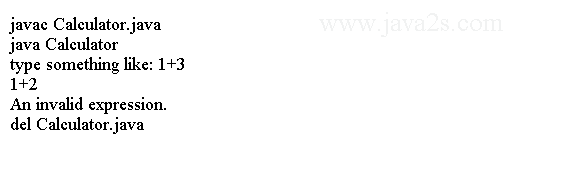# Java IO Tutorial - Java Scanner

To read numbers from the standard input, we have to read it as a string and parse it to a number.

The `Scanner` class in java.util package reads and parses a text, based on a pattern, into primitive types and strings.

The text source can be an InputStream, a file, a String object, or a Readable object.

We can use a Scanner object to read primitive type values from the standard input System.in.

The following code illustrates how to use the Scanner class by building a trivial calculator to perform addition, subtraction, multiplication, and division.

```import java.util.Scanner;
//from  ww  w  .  j a v a2  s  .c o m
public class Calculator {
public static void main(String[] args) {
System.out.println("type something like: 1+3");
Scanner scanner = new Scanner(System.in);
double n1 = Double.NaN;
double n2 = Double.NaN;
String operation = null;

try {
n1 = scanner.nextDouble();
operation = scanner.next();
n2 = scanner.nextDouble();
double result = calculate(n1, n2, operation);
System.out.printf("%s %s  %s  = %.2f%n", n1, operation, n2, result);
}

catch (Exception e) {
System.out.println("An invalid expression.");
}
}

public static double calculate(double op1, double op2, String operation) {
switch (operation) {
case "+":
return op1 + op2;
case "-":
return op1 - op2;
case "*":
return op1 * op2;
case "/":
return op1 / op2;
}

return Double.NaN;
}
}
```

The code above generates the following result.